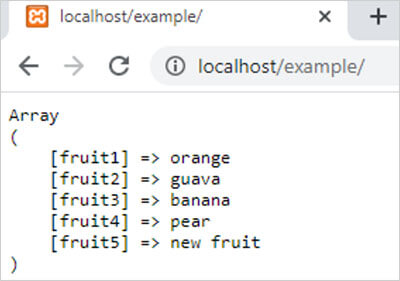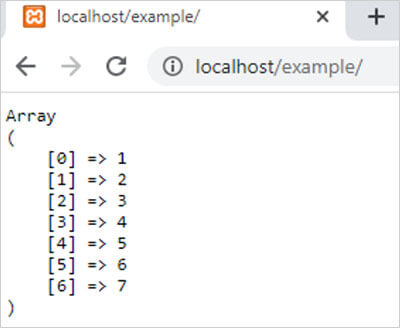array_replace() function in php || PHP Functions

array_replace() function in php

0 1085

array_replace() function of PHP is used to replace the value of one array to the value of another one or more arrays. It is an inbuilt function of PHP.

Here are some rules which explain the working of this function:

• If a key of the first array is present in the second array then this function replace the value of the first array to the value of the second array to that particular key.
• If a key of the first array not present in the second array then it does not change.
• If a key of the second array does not exist in the first array then this function insert a new item in the first array which contains the value of this key.
• In case of more than two arrays this function checks all above conditions to all arrays.

Syntax:

array_replace(\$arr1,\$arr2,....,\$arrN);

here,

\$arr1,\$arr2 and \$arrN are PHP arrays.

Example 1:

"orange","fruit2"=>"apple","fruit3"=>"banana","fr  uit4"=>"pear");
\$arr2=array("fruit2"=>"guava","fruit5"=>"new fruit");
\$result=array_replace(\$arr1,\$arr2);
echo "<pre>";
print_r(\$result);
?>

Output:Example 2:

<?php
\$arr1=array("apple","banana","pear");
\$arr2=array(1,2,3,4,5,6,7);
\$result=array_replace(\$arr1,\$arr2);
echo "<pre>";
print_r(\$result);
?>

Output:Share: